# The "Cubic Formula"

#### Introduction.Knowledge of the quadratic formula is older than the Pythagorean Theorem. Solving a cubic equation, on the other hand, was the first major success story of Renaissance mathematics in Italy. The solution was first published by Girolamo Cardano (1501-1576) in his Algebra book Ars Magna.

Our objective is to find a real root of the cubic equation

ax3+bx2+cx+d=0.

The other two roots (real or complex) can then be found by polynomial division and the quadratic formula. The solution proceeds in two steps. First, the cubic equation is "depressed"; then one solves the depressed cubic.

#### Depressing the cubic equation.This trick, which transforms the general cubic equation into a new cubic equation with missing x2-term is due to Nicolò Fontana Tartaglia (1500-1557). We apply the substitution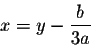to the cubic equation, to obtain: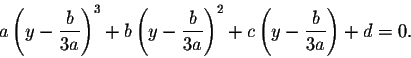Multiplying out and simplifying, we obtain the "depressed" cubic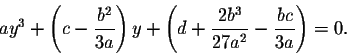Let's try this for the example

2x3-30x2+162x-350=0.

Our substitution will be x=y+5; expanding and simplifying, we obtain the depressed cubic equation

y3+6y-20=0.

#### Solving the depressed cubic.

We are left with solving a depressed cubic equation of the form

y3+Ay=B.

How to do this had been discovered earlier by Scipione del Ferro (1465-1526).

We will find s and t so that

 3st = A (1) s3-t3 = B. (2)

It turns out that y=s-t will be a solution of the depressed cubic. Let's check that: Replacing A, B and y as indicated transforms our equation into

(s-t)3+3st (s-t)=s3-t3.

This is true since we can simplify the left side by using the binomial formula to:

(s3-3s2t+3st2-t3)+(3s2t-3st2)=s3-t3.

How can we find s and t satisfying (1) and (2)? Solving the first equation for s and substituting into (2) yields: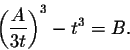Simplifying, this turns into the "tri-quadratic" equation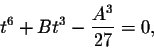which using the substitution u=t3 becomes the quadratic equation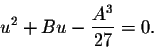From this, we can find a value for u by the quadratic formula, then obtain t, afterwards s and we're done.

Let's do the computation for our example

y3+6y=20.

We need s and t to satisfy
 3st = 6 (3) s3-t3 = 20 (4)

Solving for s in (3) and substituting the result into (4) yields: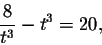which multiplied by t3 becomes

t6+20t3-8=0.

Using the quadratic formula, we obtain that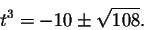We will discard the negative root, then take the cube root to obtain t: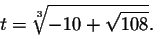By Equation (4),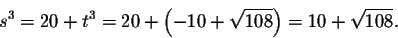Our solution y for the depressed cubic equation is the difference of s and t: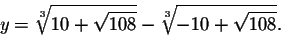The solution to our original cubic equation

2x3-30x2+162x-350=0

is given by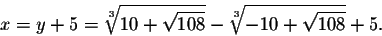#### Concluding remarks.

I will not discuss a slight problem you can encounter, if you follow the route outlined. What problem am I talking about?

Shortly after the discovery of a method to solve the cubic equation, Lodovico Ferrari (1522-1565), a student of Cardano, found a similar method to solve the quartic equation.

This section is loosely based on a chapter in the book Journey Through Genius by William Dunham.

#### Exercise 1.

Show that y=2 is a solution of our depressed cubic

y3+6y-20=0.

Then find the other two roots. Which of the roots equals our solution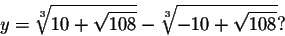#### Exercise 2.

Transform the cubic equation

x3-6x2+14x-15=0

into a depressed cubic.

#### Exercise 3.

Find a real root of the cubic equation in Exercise 2.

(This is for practice purposes only; to make the computations a little less messy, the root will turn out to be an integer, so one could use the Rational Zero test instead.)[Back] [Next: The Geometry of the Cubic Formula]
[Algebra] [Trigonometry] [Complex Variables]
[Calculus] [Differential Equations] [Matrix Algebra]S.O.S MATHematics home page

Do you need more help? Please post your question on our S.O.S. Mathematics CyberBoard.Helmut Knaust
98-05-20